# Software at CIS : lddmm-similitude and lddmm-rigid

## Algorithm

Let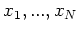andbe corresponding template and target landmarks respectively. Find the rotation,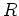, translation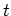, and (for lddmm-similitude) scale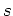which minimize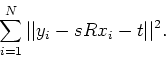For lddmm-rigid, scaleis fixed at 1.0.

## Reference

S. Umeyama, "Least-Squares Estimation of Transformation Parameters Between Two Point Patterns".IEEE Transactions on Pattern Analysis and Machine Intelligence, Vol 12, NO 4, April 1991. (pdf)

lddmm-similitude matching is a program to determine the similarity between two landmark data sets based on rotation, translation, and scale. The program will write the rotation, translation and scale to standard out and create a file representing the transformed template.

lddmm-rigid matching is a program to determine the similarity between two landmark data sets based on rotation and translation. The program will write the rotation and translation to standard out and create a file representing the transformed template.

## Typical Usage and Parameters:

lddmm-similitude || lddmm-rigid [OPTIONS] x_file y_file tx_file norm_constant [Rts_file]

The following are the command line options.

1. x_file: the template landmark file
2. y_file: the target landmark file
3. tx_file: the output file containing the transformed template. Use /dev/null if this is not required.
4. norm_constant: always use 1.0
5. Rts_file: omit this option
6. Options:
1. -r --allowReflection: allow a transform that includes a reflection
2. -a --all-info: print data provenance info
3. -x --all-info-xml: print data provenance info in an xml format
4. -v --version: print program version information
5. -h --help: print program usage information
Landmark File Format:

The format for a 2-D landmark file is as follows:
  N x 2 [ x_0 y_0 x_1 y_1 . . x_N y_N ] 

And for a 3-D file:

  N x 3 [ x_0 y_0 z_0 x_1 y_1 z_1 . . x_N y_N z_N ] 

Output File Format:

The program will format the output file based on the extension of the output file:

• bz - blitz++ (or runSim) format, described above.
• lmk - BrainWorks format.
• fcsv - Slicer 3D fiducial format.
Scripting:
Here is an example Perl script that computes the similarity for every pair of landmark sets in start_folder and writes the output line by line to a text file for later analysis. This can easily be adapted to do different comparisons (ex. left & right data separately) by calling the function do_sims on a number of different lists:
#!/usr/bin/perl
$program = "lddmm-similitude";$start_folder = "/cis/project/botteron/hippocampus/Converted_Lmk";

@inlist= ls -1 $start_folder/*.lmk;$outfile = "/cis/project/botteron/hippocampus/left_scale_list.txt";

&do_sims ($infile, \@outlist); sub do_sims { my$outfile = shift;
my $list_ref=shift; my @list = @$list_ref;
print "$outfile\n"; print "List size:$#list\n";

for($i=0;$i<=$#list;$i++) {
chomp $list[$i];
$list[$i] =~ /^.+\/(.+)/;
$shortlist[$i] = $1; } open OUT, ">$outfile";
for($i=0;$i<=$#list;$i++) {
for($j=$i+1; $j<=$#list; $j++) {$sim = $program$list[$i]$list[$j] /dev/null 1.0 | cut -f3 -d\\ ; print OUT "$shortlist[$i]:$shortlist[$j]:$sim";
}
print "Finished $shortlist[$i]\n";
}
close OUT;
}


Software developed with support from National Institutes of Health NCRR grant P41 RR15241.